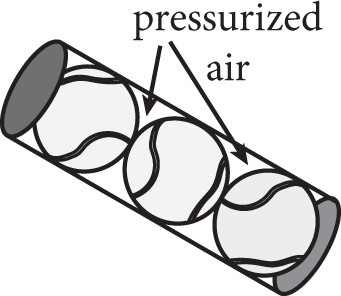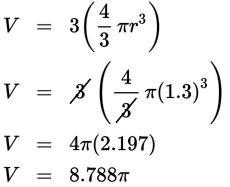# SAT Math Multiple Choice Question 447: Answer and Explanation

### Test Information

Question: 447

12.Higher-quality tennis balls are typically packaged in cylindrical cans, as shown above, which are pressurized with air to keep them fresh. If the can and the tennis balls have the same diameter, 2.6 inches, what is the volume in cubic inches of the air inside the can around the tennis balls? Assume that each tennis ball is tangent to the next and that the top and bottom tennis balls are tangent to the top and bottom of the can.

• A. 4.4π
• B. 8.1π
• C. 10.3π
• D. 29.3π

Explanation:

A

Difficulty: Hard

Strategic Advice: Don't forget to refer to the formula page on Test Day. The can is a cylinder and a tennis ball is a sphere, so you'll need to use both equations.

Getting to the Answer: Make a plan before you start plugging values into the formulas: The volume of the air is equal to the volume of the can minus the volume of the three tennis balls. For both formulas, you will need the radius. The diameters of the cylinder and the balls are the same, 2.6, but you need the radius, so divide by 2 to get 2.6 ÷ 2 = 1.3. For the cylinder you also need the height. Because there are 3 tennis balls and the top and bottom balls are tangent to the top and bottom of the can, the height is simply the diameter multiplied by 3, which is 2.6 × 3 = 7.8. Now you're ready to use the formulas. A quick peek at the answer choices will tell you that you don't need to simplify completely.

First, find the volume of the whole can:Next, find the volume of three tennis balls:Finally, subtract to get 13.182π – 8.788π = 4.394π, or about 4.4π cubic inches of air.